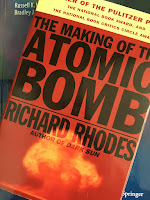Friday, March 10, 2017

My Honors College Class: The Making of the Atomic BombThe Making of the Atomic Bomb, by Richard Rhodes.
This semester I am teaching a class in Oakland University’s Honors College called “The Making of the Atomic Bomb,” based on Richard Rhodes’s book by the same name. The class is a mixture of nuclear physics, a history of the Manhattan Project, and a discussion about World War II (today we discuss Pearl Harbor). I became interested in this topic from the writings of Cameron Reed of Alma College here in Michigan.

The Honors College students are outstanding, but they are from disciplines throughout the university and do not necessarily have strong math and science backgrounds. Therefore the mathematics in this class is minimal, but nevertheless we do a two or three quantitative examples. For instance, Chadwick’s discovery of the neutron in 1932 was based on conclusions drawn from collisions of particles, and relies primarily on conservation of energy and momentum. When we analyze Chadwick’s experiment in my Honors College class, we consider the head-on collision of two particles of mass M1 and M2. Before the collision, the incoming particle M1 has kinetic energy T and the target particle M2 is at rest. After the collision, M1 has kinetic energy T1 and M2 has kinetic energy T2.

Intermediate Physics for Medicine and Biology examines an identical situation in Section 15.11 on Charged-Particle Stopping Power.
The maximum possible energy transfer Wmax can be calculated using conservation of energy and momentum. For a collision of a projectile of mass M1 and kinetic energy T with a target particle of mass M2 which is initially at rest, a nonrelativistic calculation gives
One important skill I teach my Honors College students is how to extract a physical story from a mathematical expression. One way to begin is to introduce some dimensionless parameters. Let t be the ratio of kinetic energy picked up by M2 after the collision to the incoming kinetic energy T, so t = T2/T or, using the notation in IPMB, t = Wmax/T (the subscript “max” arises because this maximum value of T2 corresponds to a head-on collision; a glancing blow will result in a smaller T2). Also, let m be the ratio of M1 to M2, so m = M1/M2. A little algebra results in the simpler-looking equation
The goal is to unmask the physical behavior hidden in this equation. The best way to proceed is to examine limiting cases. There are three that are of particular interest.

m much less than 1. When m is small (think of a fast-moving proton colliding with a stationary lead nucleus) the denominator is approximately one, so t = 4m. Because m is small, so is t. This means the proton merely bounces back elastically as if striking a brick wall. Little energy is transferred to the lead nucleus.
m much greater than 1. When m is large (think of a fast-moving lead nucleus smashing into a stationary proton) the denominator is approximately m2, so t = 4/m. Because m is large, t is small. This means the lead continues on as if the proton were not even there, with little loss of energy. The proton flies off at a high speed, but because of its small mass it carries off negligible energy.
m equal to 1. When m is one (think of a neutron colliding with a proton, which was the situation examined by Chadwick), the denominator becomes 4, and t = 1. All of the energy of the neutron is transferred to the proton. The neutron stops and the proton flies off at the same speed the neutron flew in.
A mantra I emphasize to my students is that equations are not just things you put numbers into to get other numbers. Equations tell a physical story. Being able to extract this story from an equation is one of the most important abilities a student must learn. Never pass up a chance to reinforce this skill.